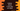# Python program to check if two sets are equal in 3 ways## Python program to check if two sets are equal:

Python `set` is an inbuilt data type and it is used to store collection of data. `set` doesn’t allow any duplicate elements and its items are unordered and un-indexed. If two sets have the same elements(in any order), these are considered equal.

This post will show you how to check if two sets are equal or not. We will learn two different ways to do that.

### Method 1: By using the `==` operator:

We can check if two sets are equal or not by using the `==` operator. It will check if two sets are equal or not and return one boolean value. It returns `True` if the elements of the sets are equal. Else, it returns `False`. The order of the elements in the set is not considered for the comparison.

For example:

``````first_set = {'one', 'two', 'three'}
second_set = {'one', 'two', 'three'}

print(first_set == second_set)``````

It will print:

``True``

Similarly, if we have different elements in the sets:

``````first_set = {'one', 'two', 'three'}
second_set = {'one', 'two', 'three', 'four'}

print(first_set == second_set)``````

It will print:

``False``

For the following example:

``````first_set = {'one', 'two', 'three', 'one'}
second_set = {'one', 'two', 'three'}

print(first_set == second_set)``````

It will print `True` because we can’t have duplicate items in a set. So, the set `{'one', 'two', 'three', 'one'}` is equal to `{'one', 'two', 'three'}`.

### Method 2: By using `!=` operator:

The operator `!=` or not equal to can be used to check if one set is not equal to another set. It will return `True` if the sets are not equal and `False` otherwise. Let’s try it with an example:

``````first_set = {'one', 'two', 'three', 'one'}
second_set = {'one', 'two', 'three'}
third_set = {'one', 'two', 'three', 'four'}

print(first_set != second_set)
print(first_set != third_set)``````

It will print `False` for the first one and `True` for the second one.

### Method 3: By using the `symmetric_difference()` method:

We can also use the `symmetric_difference` method to find the difference between two sets. The `symmetric_difference` method is defined as below:

``set1.symmetric_difference(set2)``

The `summetric_difference()` method returns one set with zero elements if both hold similar elements. Otherwise, it will return the elements which are not common in the sets. We can check the length of the returned set to find if both are equal or not. The `len()` method can be used to check the length of the returned set. The return value of this method will be 0 if the sets are equal.

Below is the complete program:

``````first_set = {'one', 'two', 'three'}
second_set = {'one', 'two', 'three', 'one'}

if len(first_set.symmetric_difference(second_set)) == 0:
print('Both sets are equal')
else:
print('Sets are not equal')``````

If you run this program, it will print the below output:

``Both sets are equal``

### Can we use the `difference()` method to check if two sets are equal or not?

We can’t use the `difference()` method to check for set equality. The syntax of the `difference` method is:

``set1.difference(set2)``

It will return the elements that are in `set1` but not in `set2`. If the `set1` is a subset of `set2`, it will return an empty set. e.g.

``````first_set = {'one', 'two', 'three'}
second_set = {'one', 'two', 'three', 'four'}

if len(first_set.difference(second_set)) == 0:
print('Both sets are equal')
else:
print('Sets are not equal')``````

Even though the sets `first_set` and `second_set` are not equal, it will print those as equal.

``Both sets are equal``